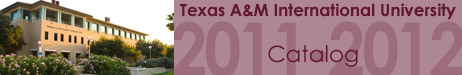Home >> Course Descriptions >> College of Arts and Sciences PHYS 4315 Mathematical Methods of Physics Three semester hours. A course presenting mathematical techniques used in physics and engineering. The course will survey, at a brief introductory level and from a physics perspective, numerous mathematical techniques from areas such as infinite series, integral transformation, applications of complex variables, matrices and tensors, special functions, partial differential equations, Green's functions, perturbation theory, integral equations, calculus of variations, and groups and group representations. Prerequisites: PHYS 2326/2126 and MATH 3330.